# JP2008005646A - Initial magnetic pole position estimating apparatus of permanent-magnet synchronous motor - Google Patents

Initial magnetic pole position estimating apparatus of permanent-magnet synchronous motor Download PDF

## Info

Publication number
JP2008005646A
JP2008005646A JP2006173785A JP2006173785A JP2008005646A JP 2008005646 A JP2008005646 A JP 2008005646A JP 2006173785 A JP2006173785 A JP 2006173785A JP 2006173785 A JP2006173785 A JP 2006173785A JP 2008005646 A JP2008005646 A JP 2008005646A
Authority
JP
Japan
Prior art keywords
phase
electrical angle
value
detection value
angle phase
Prior art date
Legal status (The legal status is an assumption and is not a legal conclusion. Google has not performed a legal analysis and makes no representation as to the accuracy of the status listed.)
Granted
Application number
JP2006173785A
Other languages
Japanese (ja)
Other versions
JP4735439B2 (en
Inventor
Katsunori Nosaka

Original Assignee
Meidensha Corp

Priority date (The priority date is an assumption and is not a legal conclusion. Google has not performed a legal analysis and makes no representation as to the accuracy of the date listed.)
Filing date
Publication date
Application filed by Meidensha Corp, 株式会社明電舎 filed Critical Meidensha Corp
Priority to JP2006173785A priority Critical patent/JP4735439B2/en
Publication of JP2008005646A publication Critical patent/JP2008005646A/en
Application granted granted Critical
Publication of JP4735439B2 publication Critical patent/JP4735439B2/en
Active legal-status Critical Current
Anticipated expiration legal-status Critical

• 230000001360 synchronised Effects 0.000 title claims abstract description 17
• 238000006243 chemical reactions Methods 0.000 claims description 32
• 238000009825 accumulation Methods 0.000 claims description 4
• 235000021171 collation Nutrition 0.000 abstract 2
• 101710063788 IDH2 Proteins 0.000 description 9
• 101710063791 IDH1 Proteins 0.000 description 8
• 238000010586 diagrams Methods 0.000 description 7
• 230000000875 corresponding Effects 0.000 description 2
• 230000003247 decreasing Effects 0.000 description 2
• 239000010410 layers Substances 0.000 description 2
• 230000001276 controlling effects Effects 0.000 description 1
• 238000007796 conventional methods Methods 0.000 description 1
• 238000004070 electrodeposition Methods 0.000 description 1
• 238000004804 winding Methods 0.000 description 1

## Images

•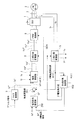•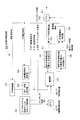•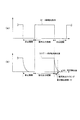•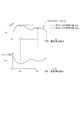•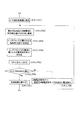•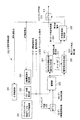•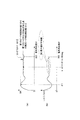•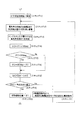•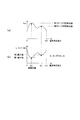•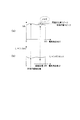•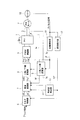## Abstract

<P>PROBLEM TO BE SOLVED: To correctly estimate an initial magnetic pole position of a permanent-magnet synchronous motor. <P>SOLUTION: A phase measurement control unit 100 successively increases the magnitude of an electric angle phase step by step. A PM motor 2 is vector-controlled by a current control unit 7 in response to a deviation between a d-axis current command Id* to be output like a pulse during a command output period and a d-axis current detection value Id(θ). The control unit 100 obtains a determination detection value as a value of the d-axis current detection value Id(θ) at a time point when the current detection period has elapsed from the completion point of the command output period. Then, the control unit 100 obtains collation data in which each electric angle phase θ is collated with each determination detection value in each electric angle phase θ. Then, the control unit 100 obtains a first electric angle at which the determination detection value is the maximum, and a second electric angle at which the determination detection value is the second maximum from the collation data; and decides the first electric angle as an initial magnetic pole position, when a difference in the determination detection value at the first and second electric angles is larger than a previously set value. <P>COPYRIGHT: (C)2008,JPO&INPIT

## Description

The present invention relates to an initial magnetic pole position estimation device for a permanent magnet type synchronous motor, and accurately estimates an initial magnetic pole position even if noise occurs or a motor has little change in inductance due to magnetic saturation. It is devised to be able to.

When performing vector control with a permanent magnet type synchronous motor (hereinafter referred to as “PM motor”) using a permanent magnet as a field source, it corresponds to the position of the magnet attached to the rotor (hereinafter referred to as “magnetic pole position”). Current control is required. Therefore, in the PM motor vector control, it is necessary to detect the magnetic pole position of the rotor.

Thus, in the current PM motor vector control apparatus, an absolute value encoder is often attached to the motor.
If the absolute encoder is not installed, the initial magnetic pole position is estimated using the harmonic current and harmonic voltage while the rotor is stopped, and the magnetic pole position is determined using the observer and induced voltage during motor rotation. Estimated driving is used.

The estimation of the initial magnetic pole position is performed using the fact that the inductance of the stator winding of the PM motor varies depending on the magnetic pole position of the rotor. That is, the electrical angle phase where the inductance becomes the smallest due to magnetic saturation is detected. Specifically, the inductance is measured while changing the electrical angle phase of the voltage generation phase for measurement, and the electrical angle phase at which the inductance is minimized is defined as the control axis (d-axis).

JP 2001-78486 A

By the way, depending on the design of the PM motor, there is a thing with little change in inductance due to magnetic saturation. Moreover, it measures using the inverter itself normally used instead of a dedicated measuring instrument. Therefore, due to the problem of product cost, a highly accurate current detector cannot be used, and there is a possibility of erroneous detection due to external noise depending on the usage environment. However, even under such conditions, it is necessary to accurately measure the inductance.

Inductance is measured by outputting a pulsed voltage and measuring the inductance based on the current response, or by controlling the current for the pulsed current command and measuring the inductance based on the magnitude of the output voltage command at that time. How to do is known.

However, in the conventional method of detecting the phase of the minimum inductance, as shown in FIG. 9, the change in the inductance L is not only the magnetic saturation, but also the difference between the d-axis inductance Ld and the q-axis inductance Lq due to the saliency. There is also a component that pulsates once between 0 to 360 ° of the electrical angle and a component that pulsates twice.

9A, the horizontal axis represents the electrical angle phase θ, and the vertical axis represents the determination detection value Idh. In FIG. 9B, the horizontal axis represents the electrical angle phase θ, and the vertical axis represents the inductance L.
The determination detection value Idh has the same meaning as that used in the embodiment of the present application. The determination detection value Idh will be briefly described. The determination detection value Idh indicates the value of the d-axis current that flows through the PM motor when a pulsed current command is input to the vector control device. Further, the inductance L is an inversely proportional calculation of the determination detection value.

In the case of FIG. 9, since the phase at which the determination detection value Idh has the maximum peak, that is, the phase at which the inductance L is the minimum peak is clear, the magnetic pole position θa at which the inductance L is the minimum peak is correctly estimated as the initial magnetic pole position. can do.

As described above, since there are a plurality of inductance change factors, in order to apply the phase estimation method based on magnetic saturation, accurate measurement cannot be performed unless there is a certain difference between the inductance at the magnetic pole position and the inductance at the counter electrode position. . In particular, a motor with little magnetic saturation may not be able to estimate the correct magnetic pole position due to the influence of noise.

For example, as shown in FIGS. 10A and 10B, when noise is generated, the electrical angle phase at which the determination detection value Idh is increased due to noise is erroneously estimated as the initial magnetic pole position.

Moreover, since magnetic saturation does not occur when the measurement current is not appropriate, there are cases where it cannot be estimated in the same way. If operation is performed using a magnetic pole position estimation value that is incorrect by 180 °, torque in the reverse direction to the torque command is generated, and an abnormality that rotates in the reverse direction occurs, which is dangerous.

An object of the present invention is to provide an initial magnetic pole position estimation device for a permanent magnet type synchronous motor that can accurately estimate the initial magnetic pole position of a PM motor.

The configuration of the present invention for solving the above problems is as follows.
A phase generator that outputs an electrical angle phase whose value gradually increases in steps from the initial value;
The command output period and the rest period are alternately set, and in the command output period, a current command setting unit that outputs a d-axis current command,
An inverter that drives a permanent magnet motor based on a three-phase voltage command;
Three-phase / two-phase output of the d-axis current detection value by converting the current detection value of the three-phase current supplied from the inverter to the permanent magnet synchronous motor using the electrical angle phase. A conversion unit;
A current control unit that outputs a q-axis voltage command and a d-axis voltage command based on a deviation between the d-axis current command and the detected d-axis current value;
A two-phase / three-phase converter that obtains a three-phase voltage command for driving the inverter by two-phase / three-phase conversion of the q-axis voltage command and the d-axis voltage command using the electrical angle phase;
A determination detection value capturing means for capturing a determination detection value that is a value of the d-axis current detection value at a time when a predetermined current detection period has elapsed from the end time of the command output period;
An accumulation unit that stores each electrical angle phase having a different value and each determination detection value when each electrical angle phase having a different value is paired and stored as reference data;
From the reference data, the first electrical angle phase at which the determination detection value is maximized, that is, the inductance is minimized is obtained, and the determination detection value at the first electrical angle phase is defined as the first peak determination detection value. A first electrical angle phase detector that
From the reference data, a second electrical angle phase at which the determination detection value is the second largest, that is, the inductance is the second smallest, is obtained, and the determination detection value at the second electrical angle phase is the second peak. A second electrical angle phase detection unit as a determination detection value;
Initial magnetic pole position output means for outputting the first electrical angle phase as the initial magnetic pole position when the absolute value of the difference between the first peak determination detection value and the second peak determination detection value is larger than a predetermined set value. When,
It is characterized by having.

The configuration of the present invention is as follows.
A phase generator that outputs an electrical angle phase whose value gradually increases in steps from the initial value;
The command output period and the rest period are alternately set, and in the command output period, a current command setting unit that outputs a d-axis current command,
An inverter that drives a permanent magnet motor based on a three-phase voltage command;
Three-phase / two-phase output of the d-axis current detection value by converting the current detection value of the three-phase current supplied from the inverter to the permanent magnet synchronous motor using the electrical angle phase. A conversion unit;
A current control unit that outputs a q-axis voltage command and a d-axis voltage command based on a deviation between the d-axis current command and the detected d-axis current value;
A two-phase / three-phase converter that obtains a three-phase voltage command for driving the inverter by two-phase / three-phase conversion of the q-axis voltage command and the d-axis voltage command using the electrical angle phase;
A determination detection value capturing means for capturing a determination detection value that is a value of the d-axis current detection value at a time when a predetermined current detection period has elapsed from the end time of the command output period;
An accumulation unit that stores each electrical angle phase having a different value and each determination detection value when each electrical angle phase having a different value is paired and stored as reference data;
From the reference data, the first electrical angle phase at which the determination detection value is maximized, that is, the inductance is minimized is obtained, and the determination detection value at the first electrical angle phase is defined as the first peak determination detection value. A first electrical angle phase detector that
The electrical angle phase that is 180 ° out of phase with the first electrical angle phase is set as the second electrical angle phase, and the determination detection value at the second electrical angle phase is referred to the second electrical angle phase with reference to the reference data. A second electrical angle phase detection unit as a value;
Initial magnetic pole position output means for outputting the first electrical angle phase as the initial magnetic pole position when the absolute value of the difference between the first peak determination detection value and the second peak determination detection value is larger than a predetermined set value. When,
It is characterized by having.

The configuration of the present invention is as follows.
The initial magnetic pole position output means includes
When the absolute value of the difference between the first peak determination detection value and the second peak determination detection value is equal to or smaller than a predetermined set value, the value of the d-axis current command set by the current command setting unit is increased to When the absolute value of the difference between the first peak determination detection value and the second peak determination detection value obtained under the d-axis current command with increased is larger than a predetermined set value, the first Is output as an initial magnetic pole position.

In the present invention, when the difference between the inductance at the electrical angle phase θ1 and the inductance at the electrical angle position θ2 opposite to the electrical angle phase θ1 (or 180 ° out of phase) is sufficiently large, Since the electrical angle phase θ1 is determined as the initial magnetic pole position, even if there are external noises, there are a plurality of inductance change factors, and the PM motor has a small inductance change due to magnetic saturation. In addition, the initial magnetic pole position can be accurately estimated.
Since the accurate magnetic pole position can be estimated in this way, it is possible to prevent the occurrence of an abnormality that occurs in the reverse direction due to the generation of torque opposite to the torque command.

The best mode for carrying out the present invention will be described below in detail based on examples.

FIG. 1 shows a magnetic pole position estimation device for a permanent magnet type synchronous motor according to Embodiment 1 of the present invention.
Prior to the description of the present invention, a control device for inverter driving the PM motor using vector control will be described with reference to FIG.

As shown in FIG. 11, the PM motor 2 rotates by supplying an equivalent three-phase current (or equivalent three-phase voltage) from the inverter 1 to the PM motor 2. The speed detector 10 (encoder) rotates with the rotor of the PM motor 2 and outputs a pulse signal P. The phase detection unit 11 obtains a phase detection value θ indicating the rotor position (phase), that is, the magnetic pole position of the PM motor 2 based on the pulse signal P, and the speed detection unit 12 obtains the PM motor based on the pulse signal P. A detected speed value ωr indicating a rotor speed of 2 is obtained. The angular velocity detection unit 13 obtains an angular velocity detection value ω from the number of poles p of the PM motor 2 and the velocity detection value ωr.

The current detection units 3 and 4 obtain u-phase and w-phase current detection values Iu and Iw. The three-phase / two-phase converter 5 obtains the v-phase current detection value Iv from the current detection values Iu, Iw, and further converts the three-phase current detection values Iu, Iv, Iw into three-phase / two-phase to detect the phase. Using the value θ, the q-axis current detection value Iq and the d-axis current detection value Id of the rotating coordinate system are obtained.

When the torque command T * is input, the torque control unit 6 uses the speed detection value ωr to obtain the d-axis current command Id * and the q-axis current command Iq * of the rotating coordinate system.

The current control unit 7 calculates a deviation (Iq * −Iq, Id * −) between the q-axis current command Iq * and the d-axis current command Id * in the rotating coordinate system and the q-axis current detection value Iq and the d-axis current detection value Id. By using a proportional / integral operator and the angular velocity ω, Id) is used to obtain the q-axis voltage command Vq * and the d-axis voltage command Vd * of the rotating coordinate system.

The two-phase / three-phase converter 8 performs coordinate conversion of the q-axis voltage command Vq * and the d-axis voltage command Vd * in the rotating coordinate system using the phase detection value θ, and the three-layer voltage command Vu * in the stationary coordinate system. Vv * and Vw * are obtained.

The PWM control unit 9 performs PWM control according to the three-phase voltage commands Vu *, Vv *, and Vw *, and the inverter 1 is controlled based on the voltage commands Vu *, Vv *, and Vw *, and the equivalent three-phase power Is supplied to the PM motor 2, and the PM motor 2 rotates.

[Description of initial magnetic pole position estimation operation]
Next, an initial magnetic pole position estimating operation by the magnetic pole position estimating device for a permanent magnet type synchronous motor according to the first embodiment of the present invention will be described with reference to FIG. When performing the operation of estimating the initial magnetic pole position, the PM motor 2 is fixed with a brake or the like so that the rotor of the PM motor 2 does not rotate.

In order to estimate the initial magnetic pole position, the phase measurement control unit 100, the current command setting unit 200, and the deviation calculation unit 201 are used. Further, the q-axis current command Iq * and the d-axis current command Id * are not output from the torque control unit 6.

The phase measurement control unit 100 has functional blocks as shown in FIG.
The 360 ° phase generation unit 102 of the phase measurement control unit 100 is controlled by the measurement control unit 101 and first sets the value of the electrical angle phase θ to 0 ° which is an initial value. The electrical angle phase θ whose value is set to 0 ° is sent to the current command setting unit 200, the three-phase / two-phase conversion unit 5, and the two-phase / three-phase conversion unit 8. The electrical angle phase θ is also transferred to the storage unit 103.

The d-axis current command setting unit 104 of the phase measurement control unit 100 sets and outputs the value of the d-axis current command Id * to a preset initial value (step S1 in FIG. 5). The d-axis current command Id * set to the initial value is sent to the current command setting unit 200.

As shown in FIG. 3A, the current command setting unit 200 keeps the value of the q-axis current command Iq * to zero (that is, does not output the q-axis current command Iq *) in all periods.
(1) During the rest period, the d-axis current command Id * is not output,
(2) In the command output period, a d-axis current command Id * having a value set by the d-axis current command setting unit 104 is output.
The rest period and the command output period are set alternately. The lengths of the pause period and the command period are set in advance. For example, the pause period is set to 2.5 to 3.0 ms, and the command period is set to 7.0 to 10 ms.

The deviation calculation unit 201 includes a d-axis current command Id * sent from the current command setting unit 200, a d-axis current detection value Id (θ = 0 °) output from the three-phase / two-phase conversion unit 5, and Deviation (Id * −Id (θ = 0 °)) is obtained and output. The d-axis current detection value Id (θ = 0 °) output from the three-phase / two-phase converter 5 will be described later.

The current control unit 7 calculates the q-axis voltage command Vq * and the d-axis voltage command Vd * of the rotating coordinate system by performing a proportional / integral calculation on the deviation (Id * −Id (θ = 0 °)). The two-phase / three-phase conversion unit 8 performs coordinate conversion of the q-axis voltage command Vq * and the d-axis voltage command Vd * in the rotating coordinate system using the electrical angle phase θ (= 0 °), and performs three-dimensional conversion in the stationary coordinate system. Phase voltage commands Vu *, Vv *, Vw * are obtained.

The PWM control unit 9 performs PWM control according to the voltage commands Vu *, Vv *, Vw *, and the inverter 1 adjusts the phase according to the voltage commands Vu *, Vv *, Vw *, and performs PWM control and The equivalent three-phase power that has undergone phase adjustment is supplied to the PM motor 2.

The current detection units 3 and 4 obtain u-phase and w-phase current detection values Iu and Iw.
The three-phase / two-phase conversion unit 5 obtains a v-phase current detection value Iv from the current detection values Iu, Iw, and further converts the three-phase current detection values Iu, Iv, Iw into a three-phase / two-phase conversion to obtain an electrical angle. Using the phase θ (= 0 °), the d-axis current detection value Id (θ = 0 °) of the rotating coordinate system when the electrical angle phase θ is 0 ° is obtained.

This d-axis current detection value Id (θ = 0 °) is sent to the deviation calculation unit 201 and the phase measurement control unit 100. As described above, the deviation calculation unit 201 calculates a deviation between the d-axis current command Id * and the detected d-axis current value Id (θ = 0 °).

As shown in FIG. 3B, the d-axis current detection value Id (θ = 0 °) is a constant value corresponding to the d-axis current command Id * during the command output period, and gradually decreases during the pause period. To do. The decreasing rate of the detected d-axis current value Id (θ) during this idle period is determined by the reactance value of the PM motor 2 (this value varies depending on the value of the electrical angle phase θ).

In the phase measurement control unit 100 to which the d-axis current detection value Id (θ = 0 °) is input, the switching means 106 determines in advance from the end of the command output period under the control of the gate control current sample timing control unit 105. When the current detection period T2 has elapsed, it is instantaneously turned on. Therefore, the determination detection value Idh that is the value of the d-axis current detection value Id (θ = 0 °) at the time when the switching means 106 is turned on is captured (see FIG. 3B).
This determination detection value Idh is sent to the storage unit 103.

For this reason, the storage unit 103 stores the electrical angle phase θ = 0 ° and the determination detection value Idh (θ = 0 °) when θ = 0 ° as a pair (step S2 in FIG. 5).

Note that the gate control current sample timing control unit 105 sends a gate control command GEN to the PWM control unit 9 in order to output a voltage command from the PWM control unit 9 at the timing shown in FIG.

Next, the 360 ° phase generation unit 102 of the phase measurement control unit 100 is controlled by the measurement control unit 101 to set the value of the electrical angle phase θ to 1 °. The electrical angle phase θ whose value is set to 1 ° is sent to the current command setting unit 200, the three-phase / two-phase conversion unit 5, and the two-phase / three-phase conversion unit 8. In addition, the electrical angle phase θ set to 1 ° is also transferred to the storage unit 103.

When the electrical angle phase θ is set to 1 ° in this way, in the phase measurement control unit 100, the gate control current sample timing control unit 105 and the switching means 106 perform the same operation as when the electrical angle phase θ is 0 °. , Θ = 1 °, and the detected detection value Idh (θ = 1 °) is taken in. Therefore, the storage unit 103 stores the electrical angle phase θ = 1 ° and the determination detection value Idh (θ = 1 °) when θ = 1 ° as a pair (step S2 in FIG. 5). ).

Thereafter, under the control of the measurement control unit 101, the value of the electrical angle phase θ set by the 360 ° phase generation unit 102 is sequentially increased in steps of 1 °, and the same as described above for each electrical angle phase. Repeat the operation.
As a result, the storage unit 103 stores the electrical angle phases (θ = 0 ° to 359 °) and the determination detection values Idh (θ = 0 °) to Idh (θ = 359 °) when the electrical angle phases are set. Is stored as a pair of control data (step S2 in FIG. 5).

The electrical angle phases (θ = 0 ° to 359 °) stored in the storage unit 103, and the determination detection values Idh (θ = 0 °) to Idh (θ = 359 °) when the electrical angle phases are set. The control data in which is paired is, for example, as shown in FIG.
The inductance of the PM motor 2 is an inversely proportional calculation of the determination detection value Idh. That is, there is a relationship of inductance L∝ (1 / Idh). Therefore, the relationship between the inductance L and the electrical angle phase θ is as shown in FIG.

The first electrical angle phase detection unit 107 of the phase measurement control unit 100 receives the reference data from the storage unit 103, for example, associating the relationship between the electrical angle phase θ and the determination detection value Idh as shown in FIG. 4 is generated, and relationship data indicating the relationship between the electrical angle phase θ and the inductance L as shown in FIG.
Then, the electrical angle phase detection unit 107 determines from the data shown in FIGS. 4A and 4B the electrical angle phase θ1 at which the inductance L is the smallest, and the value of the determination detection value Idh at this electrical angle phase θ1. The first peak determination detection value Idh1 is obtained (step S3 in FIG. 5).

The second electrical angle phase detection unit 108 of the phase measurement control unit 100 receives the reference data from the storage unit 103 in which the relationship between the electrical angle phase θ and the determination detection value Idh as shown in FIG. 4 is generated, and relationship data indicating the relationship between the electrical angle phase θ and the inductance L as shown in FIG.
Then, the electrical angle phase detection unit 108 determines from the data shown in FIGS. 4 (a) and 4 (b) that the inductance L is the next smallest (second smallest) electrical angle phase θ2, and this electrical angle phase. A second peak determination detection value Idh2 that is the value of the determination detection value Idh at the time of θ2 is obtained (step S4 in FIG. 5).

The measurement difference detection / determination unit 109 obtains a determination detection value difference Idh3 that is an absolute value of the difference between the first peak determination detection value Idh1 and the second peak determination detection value Idh2 (step S4 in FIG. 5), and this determination detection. It is determined whether or not the value difference Idh3 is larger than a predetermined set value α (step S5 in FIG. 5).

When the determination detection value difference Idh3 is larger than the set value α (step S6 in FIG. 5), the measurement difference detection / determination unit 109 turns on the switching means 110 and outputs the electrical angle phase θ1 as the initial magnetic pole position (FIG. 5). 5 step S7).

On the other hand, when the determination detection value difference Idh3 is equal to or smaller than the set value α (step S6 in FIG. 5), the measurement difference detection / determination unit 109 keeps the switching unit 110 open and does not output the electrical angle phase θ1.
In this case, the measurement difference detection / determination unit 109 outputs a re-measurement command to the measurement control unit 101 and a command to increase and correct the value of the d-axis current command to the d-axis current command setting unit 104. Is output (step S8 in FIG. 5).

As described above, when the determination detection value difference Idh3 is equal to or smaller than the set value α, the d-axis current command setting unit 104 sets the value of the predetermined current command value as the initial d-axis current command value (initial value). The added d-axis current command Id * is output.

Then, the above-described operation is performed again while the value of the d-axis current command Id * is increased.
That is, the determination detection value Idh (θ) in each electrical angle phase θ is obtained while sequentially increasing the electrical angle phase θ value generated by the 360 ° phase generation unit 102 from 0 ° by 1 °, and each electrical angle phase is obtained. Control data is created by pairing (θ = 0 ° to 359 °) with determination detection values Idh (θ = 0 °) to Idh (θ = 359 °) in the case of each electrical angle phase.
Furthermore, the peak determination detection values Idh1 and Idh2 are obtained by the electrical angle phase detection units 107 and 108, and if the determination detection value difference Idh3 is larger than the set value α, the measurement difference detection / determination unit 109 switches the switching means 110. The electrical angle phase θ1 at this time is output as the initial magnetic pole position.

Even when the value of the d-axis current command Id * is increased only once and the above processing is performed, if the determination detection value difference Idh3 is less than or equal to the set value α, the value of the previous d-axis current command ( The d-axis current command Id *, which is a value obtained by further adding a predetermined current command value to the initial value (corrected once increase), is output, and the above operation is repeated.

Then, if the series of operations for performing the above-described processing by increasing the value of the d-axis current command Id * is repeated, the determination detection value difference Idh3 will eventually become larger than the set value α. The determination unit 109 inputs the switching means 110 and outputs the electrical angle phase θ1 at that time as the initial magnetic pole position.

As described above, in the first embodiment, the peak determination detection value Idh1 at the electrical angle phase θ1 at which the inductance L is minimized, and the peak at the electrical angle phase θ2 at which the inductance L is the next smallest (second smallest). When the difference from the determination detection value Idh2 is sufficiently large, in other words, the difference between the inductance at the electrical angle phase θ1 and the inductance at the electrical angle position θ2 that is opposite to the electrical angle phase θ1 is sufficiently large. In this case, the electrical angle phase θ1 is estimated as the initial magnetic pole position.

As described above, when the difference between the inductance at the electrical angle phase θ1 and the inductance at the electrical angle position θ2 that is opposite to the electrical angle phase θ1 is sufficiently large, the electrical angle phase θ1 is set to the initial magnetic pole position. Therefore, even if there are external noises, there are multiple inductance change factors, and even PM motors with little inductance change due to magnetic saturation, the initial magnetic pole position can be accurately determined. Can be estimated.
Since the accurate magnetic pole position can be estimated in this way, it is possible to prevent the occurrence of an abnormality that occurs in the reverse direction due to the generation of torque opposite to the torque command.

Next, a description will be given of a magnetic pole position estimation device for a permanent magnet synchronous motor according to a second embodiment of the present invention. In the second embodiment, a phase measurement control unit 300 shown in FIG. 6 is used instead of the phase measurement control unit 100 shown in FIG.

Here, prior to the description of the second embodiment, a control device for inverter driving the PM motor using vector control will be described with reference to FIG.

As shown in FIG. 11, the PM motor 2 rotates by supplying an equivalent three-phase current (or equivalent three-phase voltage) from the inverter 1 to the PM motor 2. The speed detector 10 (encoder) rotates with the rotor of the PM motor 2 and outputs a pulse signal P. The phase detection unit 11 obtains a phase detection value θ indicating the rotor position (phase), that is, the magnetic pole position of the PM motor 2 based on the pulse signal P, and the speed detection unit 12 obtains the PM motor based on the pulse signal P. A detected speed value ωr indicating a rotor speed of 2 is obtained. The angular velocity detection unit 13 obtains an angular velocity detection value ω from the number of poles p of the PM motor 2 and the velocity detection value ωr.

The current detection units 3 and 4 obtain u-phase and w-phase current detection values Iu and Iw. The three-phase / two-phase converter 5 obtains the v-phase current detection value Iv from the current detection values Iu, Iw, and further converts the three-phase current detection values Iu, Iv, Iw into three-phase / two-phase to detect the phase. Using the value θ, the q-axis current detection value Iq and the d-axis current detection value Id of the rotating coordinate system are obtained.

When the torque command T * is input, the torque control unit 6 uses the speed detection value ωr to obtain the d-axis current command Id * and the q-axis current command Iq * of the rotating coordinate system.

The current control unit 7 calculates a deviation (Iq * −Iq, Id * −) between the q-axis current command Iq * and the d-axis current command Id * in the rotating coordinate system and the q-axis current detection value Iq and the d-axis current detection value Id. By using a proportional / integral operator and the angular velocity ω, Id) is used to obtain the q-axis voltage command Vq * and the d-axis voltage command Vd * of the rotating coordinate system.

The two-phase / three-phase converter 8 performs coordinate conversion of the q-axis voltage command Vq * and the d-axis voltage command Vd * in the rotating coordinate system using the phase detection value θ, and the three-layer voltage command Vu * in the stationary coordinate system. Vv * and Vw * are obtained.

The PWM control unit 9 performs PWM control according to the three-phase voltage commands Vu *, Vv *, and Vw *, and the inverter 1 is controlled based on the voltage commands Vu *, Vv *, and Vw *, and the equivalent three-phase power Is supplied to the PM motor 2, and the PM motor 2 rotates.

[Description of initial magnetic pole position estimation operation]
Next, an initial magnetic pole position estimating operation by the magnetic pole position estimating device for a permanent magnet type synchronous motor according to the second embodiment of the present invention will be described with reference to FIG. When performing the operation of estimating the initial magnetic pole position, the PM motor 2 is fixed with a brake or the like so that the rotor of the PM motor 2 does not rotate.

In order to estimate the initial magnetic pole position, a phase measurement control unit 300 (see FIG. 6), a current command setting unit 200, and a deviation calculation unit 201 are used. Further, the q-axis current command Iq * and the d-axis current command Id * are not output from the torque control unit 6.

The phase measurement control unit 300 has functional blocks as shown in FIG.
The 360 ° phase generation unit 302 of the phase measurement control unit 300 is controlled by the measurement control unit 301 to first set the value of the electrical angle phase θ to an initial value of 0 °. The electrical angle phase θ whose value is set to 0 ° is sent to the current command setting unit 200, the three-phase / two-phase conversion unit 5, and the two-phase / three-phase conversion unit 8. The electrical angle phase θ is also transferred to the storage unit 303.

The d-axis current command setting unit 304 of the phase measurement control unit 300 sets the value of the d-axis current command Id * to a preset initial value and outputs it (step S11 in FIG. 8). The d-axis current command Id * set to the initial value is sent to the current command setting unit 200.

As shown in FIG. 3A, the current command setting unit 200 keeps the value of the q-axis current command Iq * to zero (that is, does not output the q-axis current command Iq *) in all periods.
(1) During the rest period, the d-axis current command Id * is not output,
(2) In the command output period, a d-axis current command Id * having a value set by the d-axis current command setting unit 104 is output.
The rest period and the command output period are set alternately. The lengths of the pause period and the command period are set in advance. For example, the pause period is set to 2.5 to 3.0 ms, and the command period is set to 7.0 to 10 ms.

The deviation calculation unit 201 includes a d-axis current command Id * sent from the current command setting unit 200, a d-axis current detection value Id (θ = 0 °) output from the three-phase / two-phase conversion unit 5, and Deviation (Id * −Id (θ = 0 °)) is obtained and output. The d-axis current detection value Id (θ = 0 °) output from the three-phase / two-phase converter 5 will be described later.

The current control unit 7 calculates the q-axis voltage command Vq * and the d-axis voltage command Vd * of the rotating coordinate system by performing a proportional / integral calculation on the deviation (Id * −Id (θ = 0 °)). The two-phase / three-phase conversion unit 8 performs coordinate conversion of the q-axis voltage command Vq * and the d-axis voltage command Vd * in the rotating coordinate system using the electrical angle phase θ (= 0 °), and performs three-dimensional conversion in the stationary coordinate system. Phase voltage commands Vu *, Vv *, Vw * are obtained.

The PWM control unit 9 performs PWM control according to the voltage commands Vu *, Vv *, Vw *, and the inverter 1 adjusts the phase according to the voltage commands Vu *, Vv *, Vw *, and performs PWM control and The equivalent three-phase power that has undergone phase adjustment is supplied to the PM motor 2.

The current detection units 3 and 4 obtain u-phase and w-phase current detection values Iu and Iw.
The three-phase / two-phase conversion unit 5 obtains a v-phase current detection value Iv from the current detection values Iu, Iw, and further converts the three-phase current detection values Iu, Iv, Iw into a three-phase / two-phase conversion to obtain an electrical angle. Using the phase θ (= 0 °), the d-axis current detection value Id (θ = 0 °) of the rotating coordinate system when the electrical angle phase θ is 0 ° is obtained.

This d-axis current detection value Id (θ = 0 °) is sent to the deviation calculation unit 201 and the phase measurement control unit 300. As described above, the deviation calculation unit 201 calculates a deviation between the d-axis current command Id * and the detected d-axis current value Id (θ = 0 °).

As shown in FIG. 3B, the d-axis current detection value Id (θ = 0 °) is a constant value corresponding to the d-axis current command Id * during the command output period, and gradually decreases during the pause period. To do. The decreasing rate of the detected d-axis current value Id (θ) during this idle period is determined by the reactance value of the PM motor 2 (this value varies depending on the value of the electrical angle phase θ).

In the phase measurement control unit 300 to which the d-axis current detection value Id (θ = 0 °) is input, the switching unit 306 determines in advance from the end of the command output period under the control of the gate control current sample timing control unit 305. When the current detection period T2 has elapsed, it is instantaneously turned on. Therefore, the determination detection value Idh that is the value of the d-axis current detection value Id (θ = 0 °) at the time when the switching unit 306 is turned on is taken in (see FIG. 3B).
This determination detection value Idh is sent to the storage unit 303.

For this reason, the storage unit 303 stores the electrical angle phase θ = 0 ° and the determination detection value Idh (θ = 0 °) when θ = 0 ° as a pair (step S12 in FIG. 8).

Note that the gate control current sample timing control unit 305 sends a gate control command GEN to the PWM control unit 9 in order to output a voltage command from the PWM control unit 9 at the timing shown in FIG.

Next, the 360 ° phase generation unit 302 of the phase measurement control unit 300 is controlled by the measurement control unit 301 to set the value of the electrical angle phase θ to 1 °. The electrical angle phase θ whose value is set to 1 ° is sent to the current command setting unit 200, the three-phase / two-phase conversion unit 5, and the two-phase / three-phase conversion unit 8. Further, the electrical angle phase θ set to 1 ° is also transferred to the storage unit 303.

When the electrical angle phase θ is set to 1 ° in this way, in the phase measurement control unit 300, the gate control current sample timing control unit 305 and the switching means 306 perform the same operation as when the electrical angle phase θ is 0 °. , Θ = 1 °, and the detected detection value Idh (θ = 1 °) is taken in. Therefore, the storage unit 303 stores the electrical angle phase θ = 1 ° and the determination detection value Idh (θ = 1 °) when θ = 1 ° as a pair (step S12 in FIG. 8). ).

Thereafter, under the control of the measurement control unit 301, the value of the electrical angle phase θ set by the 360 ° phase generation unit 302 is sequentially increased step by step by 1 °, and the same as described above for each electrical angle phase. Repeat the operation.
As a result, the storage unit 303 stores the electrical angle phases (θ = 0 ° to 359 °) and the determination detection values Idh (θ = 0 °) to Idh (θ = 359 °) when the electrical angle phases are set. The control data paired with is accumulated (step S12 in FIG. 8).

The electrical angle phases (θ = 0 ° to 359 °) stored in the storage unit 303 and the determination detection values Idh (θ = 0 °) to Idh (θ = 359 °) when the electrical angle phases are set. The control data in which is paired is, for example, as shown in FIG.
The inductance of the PM motor 2 is an inversely proportional calculation of the determination detection value Idh. That is, there is a relationship of inductance L∝ (1 / Idh). Therefore, the relationship between the inductance L and the electrical angle phase θ is as shown in FIG.

The first electrical angle phase detection unit 307 of the phase measurement control unit 300 receives the reference data from the storage unit 303 in which the relationship between the electrical angle phase θ and the determination detection value Idh as shown in FIG. And the relational data indicating the relation between the electrical angle phase θ and the inductance L as shown in FIG.
Then, the electrical angle phase detection unit 307 determines, based on the data shown in FIGS. 7A and 7B, the electrical angle phase θ1 at which the inductance L is the smallest, and the value of the determination detection value Idh at the time of this electrical angle phase θ1. The first peak determination detection value Idh1 is obtained (step S13 in FIG. 8).

In the example of the second embodiment, the electrical angle phase at which the inductance L is the second smallest is unclear. This point is different from the first embodiment.
Thus, in the second embodiment, the electrical angle phase θ2 obtained by the electrical angle phase detection unit 307 is opposite to the electrical angle phase θ1 having the smallest inductance L (at a position that is 180 ° out of phase from θ1). Is calculated by the electrical angle phase calculation unit 311.

Specifically, as shown in steps S14 to S16 in FIG. 8, the calculation of θ2 = θ1 + 180 ° is performed (step S14), and θ2 obtained by this calculation is 360 ° or less (step S15). Let θ2 calculated in step S14 be the electrical angle of the counter electrode of θ1.
Further, when θ2 obtained by the calculation in step S14 exceeds 360 ° (step S15), in step S16, θ2 = 360 ° −θ2 is calculated, and the calculation is performed in step S16. Θ2 is the electrical angle of the counter electrode of θ1.

The second electrical angle phase detection unit 308 of the phase measurement control unit 300 receives the reference data from the storage unit 303 in which the relationship between the electrical angle phase θ and the determination detection value Idh as shown in FIG. And the relational data indicating the relation between the electrical angle phase θ and the inductance L as shown in FIG.
Then, the electrical angle phase detection unit 308 takes in the electrical angle phase θ2 calculated by the electrical angle phase calculation unit 311 and sets the value of the determination detection value Idh at the time of the electrical angle phase θ2 as the second peak determination detection value Idh2. Ask.
That is, when the electrical angle phase θ2, as shown in FIG. 7A, the determination detection value Idh does not have a peak characteristic, but when the electrical angle phase θ2, the determination detection value Idh is regarded as the second peak. Thus, the second peak determination detection value Idh2 is obtained.

The measurement difference detection / determination unit 309 obtains a determination detection value difference Idh3 that is an absolute value of the difference between the first peak determination detection value Idh1 and the second peak determination detection value Idh2 (step S18 in FIG. 8), and this determination detection. It is determined whether or not the value difference Idh3 is larger than a predetermined set value α (step S19 in FIG. 8).

When the determination detection value difference Idh3 is larger than the set value α (step S19 in FIG. 8), the measurement difference detection / determination unit 309 turns on the switching means 310 and outputs the electrical angle phase θ1 as the initial magnetic pole position (FIG. 8). 8 step S20).

On the other hand, when the determination detection value difference Idh3 is equal to or smaller than the set value α (step S19 in FIG. 8), the measurement difference detection / determination unit 309 keeps the switching unit 310 open and does not output the electrical angle phase θ1.
In this case, the measurement difference detection / determination unit 309 outputs a re-measurement command to the measurement control unit 301 and a command to increase and correct the value of the d-axis current command to the d-axis current command setting unit 304. Is output (step S21 in FIG. 8).

As described above, when the determination detection value difference Idh3 is equal to or smaller than the set value α, the d-axis current command setting unit 304 sets the value of the current command value determined in advance to the value (initial value) of the first d-axis current command. The added d-axis current command Id * is output.

Then, the above-described operation is performed again while the value of the d-axis current command Id * is increased.
That is, the determination detection value Idh (θ) in each electrical angle phase θ is obtained while sequentially increasing the value of the electrical angle phase θ generated by the 360 ° phase generator 302 from 0 ° by 1 °, and each electrical angle phase Control data is created by pairing (θ = 0 ° to 359 °) with determination detection values Idh (θ = 0 °) to Idh (θ = 359 °) in the case of each electrical angle phase.
Furthermore, the peak determination detection values Idh1 and Idh2 are obtained by the electrical angle phase detection units 307 and 308. If the determination detection value difference Idh3 is larger than the set value α, the measurement difference detection / determination unit 309 switches the switching means 310. The electrical angle phase θ1 at this time is output as the initial magnetic pole position.

Even when the value of the d-axis current command Id * is increased only once and the above processing is performed, if the determination detection value difference Idh3 is less than or equal to the set value α, the value of the previous d-axis current command ( The d-axis current command Id *, which is a value obtained by further adding a predetermined current command value to the initial value (corrected once increase), is output, and the above operation is repeated.

Then, if the series of operations for performing the above-described processing by increasing the value of the d-axis current command Id * is repeated, the determination detection value difference Idh3 will eventually become larger than the set value α. The determination unit 309 inputs the switching unit 310 and outputs the electrical angle phase θ1 at that time as the initial magnetic pole position.

Thus, in the second embodiment, the peak determination detection value Idh1 at the electrical angle phase θ1 at which the inductance L is minimum, and the peak determination detection value Idh2 at the electrical angle phase θ2 that is 180 ° out of phase with the electrical angle phase θ1. In other words, when the difference between the inductance at the electrical angle phase θ1 and the inductance at the electrical angle position θ2 opposite to the electrical angle phase θ1 is sufficiently large, The angular phase θ1 is estimated as the initial magnetic pole position.

As described above, when the difference between the inductance at the electrical angle phase θ1 and the inductance at the electrical angle position θ2 that is opposite to the electrical angle phase θ1 is sufficiently large, the electrical angle phase θ1 is set to the initial magnetic pole position. Therefore, even if there are external noises, there are multiple inductance change factors, and even PM motors with little inductance change due to magnetic saturation, the initial magnetic pole position can be accurately determined. Can be estimated.
Since the accurate magnetic pole position can be estimated in this way, it is possible to prevent the occurrence of an abnormality that occurs in the reverse direction due to the generation of torque opposite to the torque command.

In addition, the initial magnetic pole position can be accurately estimated even when there is no peak in the determination detection value at the electrical angle phase θ2.

The block block diagram which shows the vector control apparatus to which this invention is applied. The block block diagram which shows the phase measurement control part used in Example 1 of this invention. FIG. 3 is a characteristic diagram showing a d-axis current command and a d-axis current detection value in the first embodiment. FIG. 3 is a characteristic diagram showing a difference between a detected detection value and an inductance in Example 1. FIG. 3 is a flowchart showing the operation of the first embodiment. The block block diagram which shows the phase measurement control part used in Example 2 of this invention. FIG. 10 is a characteristic diagram showing a difference between detected detection values and inductance in Example 2. FIG. 9 is a flowchart showing the operation of the second embodiment. The characteristic view which shows the determination detection value and inductance in a prior art example. The characteristic view which shows the determination detection value and inductance in a prior art example. The block block diagram which shows a vector control apparatus.

Explanation of symbols

DESCRIPTION OF SYMBOLS 1 Inverter 2 PM motor 3, 4 Current detection part 5 Three phase / two phase conversion part 6 Torque control part 7 Current control part 8 Two phase / three phase conversion part 9 PWM control part 100,300 Phase measurement control part 200 Current command setting Part

## Claims (3)

1. A phase generator that outputs an electrical angle phase whose value gradually increases in steps from the initial value;
The command output period and the rest period are alternately set, and in the command output period, a current command setting unit that outputs a d-axis current command,
An inverter that drives a permanent magnet motor based on a three-phase voltage command;
Three-phase / two-phase output of the d-axis current detection value by converting the current detection value of the three-phase current supplied from the inverter to the permanent magnet synchronous motor using the electrical angle phase. A conversion unit;
A current control unit that outputs a q-axis voltage command and a d-axis voltage command based on a deviation between the d-axis current command and the detected d-axis current value;
A two-phase / three-phase converter that obtains a three-phase voltage command for driving the inverter by two-phase / three-phase conversion of the q-axis voltage command and the d-axis voltage command using the electrical angle phase;
A determination detection value capturing means for capturing a determination detection value that is a value of the d-axis current detection value at a time when a predetermined current detection period has elapsed from the end time of the command output period;
An accumulation unit that stores each electrical angle phase having a different value and each determination detection value when each electrical angle phase having a different value is paired and stored as reference data;
From the reference data, the first electrical angle phase at which the determination detection value is maximized, that is, the inductance is minimized is obtained, and the determination detection value at the first electrical angle phase is defined as the first peak determination detection value. A first electrical angle phase detector that
From the reference data, a second electrical angle phase at which the determination detection value is the second largest, that is, the inductance is the second smallest, is obtained, and the determination detection value at the second electrical angle phase is the second peak. A second electrical angle phase detection unit as a determination detection value;
Initial magnetic pole position output means for outputting the first electrical angle phase as the initial magnetic pole position when the absolute value of the difference between the first peak determination detection value and the second peak determination detection value is larger than a predetermined set value. When,
A device for estimating an initial magnetic pole position of a permanent magnet type synchronous motor.
2. A phase generator that outputs an electrical angle phase whose value gradually increases in steps from the initial value;
The command output period and the rest period are alternately set, and in the command output period, a current command setting unit that outputs a d-axis current command,
An inverter that drives a permanent magnet motor based on a three-phase voltage command;
Three-phase / two-phase output of the d-axis current detection value by converting the current detection value of the three-phase current supplied from the inverter to the permanent magnet synchronous motor using the electrical angle phase. A conversion unit;
A current control unit that outputs a q-axis voltage command and a d-axis voltage command based on a deviation between the d-axis current command and the detected d-axis current value;
A two-phase / three-phase converter that obtains a three-phase voltage command for driving the inverter by two-phase / three-phase conversion of the q-axis voltage command and the d-axis voltage command using the electrical angle phase;
A determination detection value capturing means for capturing a determination detection value that is a value of the d-axis current detection value at a time when a predetermined current detection period has elapsed from the end time of the command output period;
An accumulation unit that stores each electrical angle phase having a different value and each determination detection value when each electrical angle phase having a different value is paired and stored as reference data;
From the reference data, the first electrical angle phase at which the determination detection value is maximized, that is, the inductance is minimized is obtained, and the determination detection value at the first electrical angle phase is defined as the first peak determination detection value. A first electrical angle phase detector that
The electrical angle phase that is 180 ° out of phase with the first electrical angle phase is set as the second electrical angle phase, and the determination detection value at the second electrical angle phase is referred to the second electrical angle phase with reference to the reference data. A second electrical angle phase detection unit as a value;
Initial magnetic pole position output means for outputting the first electrical angle phase as the initial magnetic pole position when the absolute value of the difference between the first peak determination detection value and the second peak determination detection value is larger than a predetermined set value. When,
A device for estimating an initial magnetic pole position of a permanent magnet type synchronous motor.
3. The initial magnetic pole position output means according to claim 1 or 2,
When the absolute value of the difference between the first peak determination detection value and the second peak determination detection value is equal to or smaller than a predetermined set value, the value of the d-axis current command set by the current command setting unit is increased to If the absolute value of the difference between the first peak determination detection value and the second peak determination detection value obtained under the d-axis current command with increased is greater than a predetermined set value, the first An initial magnetic pole position estimation device for a permanent magnet type synchronous motor, wherein the electrical angle phase of the permanent magnet synchronous motor is output as an initial magnetic pole position.
JP2006173785A 2006-06-23 2006-06-23 Initial magnetic pole position estimation device for permanent magnet type synchronous motor Active JP4735439B2 (en)

## Priority Applications (1)

Application Number Priority Date Filing Date Title
JP2006173785A JP4735439B2 (en) 2006-06-23 2006-06-23 Initial magnetic pole position estimation device for permanent magnet type synchronous motor

## Applications Claiming Priority (1)

Application Number Priority Date Filing Date Title
JP2006173785A JP4735439B2 (en) 2006-06-23 2006-06-23 Initial magnetic pole position estimation device for permanent magnet type synchronous motor

## Publications (2)

Publication Number Publication Date
JP2008005646A true JP2008005646A (en) 2008-01-10
JP4735439B2 JP4735439B2 (en) 2011-07-27

# Family

## Family Applications (1)

Application Number Title Priority Date Filing Date
JP2006173785A Active JP4735439B2 (en) 2006-06-23 2006-06-23 Initial magnetic pole position estimation device for permanent magnet type synchronous motor

## Country Status (1)

JP (1) JP4735439B2 (en)

## Cited By (4)

* Cited by examiner, † Cited by third party
Publication number Priority date Publication date Assignee Title
JP2010119227A (en) * 2008-11-13 2010-05-27 Honda Motor Co Ltd Phase current estimation apparatus of electric motor, and magnetic pole position estimation apparatus of electric motor
JP2011001879A (en) * 2009-06-18 2011-01-06 Toyota Motor Corp Engine starting device
JP2013500697A (en) * 2009-07-27 2013-01-07 ピルツ ゲーエムベーハー アンド コー．カーゲー Method and apparatus for fail-safe monitoring of motion variables of electric drive
CN103427733A (en) * 2012-05-21 2013-12-04 发那科株式会社 Magnetic pole position detecting device

## Families Citing this family (1)

* Cited by examiner, † Cited by third party
Publication number Priority date Publication date Assignee Title
CN107329101B (en) * 2017-07-12 2020-06-16 日立电梯（中国）有限公司 Method and system for correcting magnetic pole codes of permanent magnet synchronous traction machine

## Citations (3)

* Cited by examiner, † Cited by third party
Publication number Priority date Publication date Assignee Title
JPH07177788A (en) * 1993-10-26 1995-07-14 Toyota Motor Corp Electrical angle detector and driver of synchronous motor using it
JPH09238495A (en) * 1996-02-29 1997-09-09 Toyota Motor Corp Electric angle detecting device and driving gear for synchronous motor
JP2001078486A (en) * 1999-09-06 2001-03-23 Meidensha Corp Vector controller of permanent magnet system of synchronous motor

## Patent Citations (3)

* Cited by examiner, † Cited by third party
Publication number Priority date Publication date Assignee Title
JPH07177788A (en) * 1993-10-26 1995-07-14 Toyota Motor Corp Electrical angle detector and driver of synchronous motor using it
JPH09238495A (en) * 1996-02-29 1997-09-09 Toyota Motor Corp Electric angle detecting device and driving gear for synchronous motor
JP2001078486A (en) * 1999-09-06 2001-03-23 Meidensha Corp Vector controller of permanent magnet system of synchronous motor

## Cited By (5)

* Cited by examiner, † Cited by third party
Publication number Priority date Publication date Assignee Title
JP2010119227A (en) * 2008-11-13 2010-05-27 Honda Motor Co Ltd Phase current estimation apparatus of electric motor, and magnetic pole position estimation apparatus of electric motor
JP2011001879A (en) * 2009-06-18 2011-01-06 Toyota Motor Corp Engine starting device
JP2013500697A (en) * 2009-07-27 2013-01-07 ピルツ ゲーエムベーハー アンド コー．カーゲー Method and apparatus for fail-safe monitoring of motion variables of electric drive
CN103427733A (en) * 2012-05-21 2013-12-04 发那科株式会社 Magnetic pole position detecting device
US8766586B2 (en) 2012-05-21 2014-07-01 Fanuc Corporation Magnetic pole position detecting device for detecting magnetic pole position of rotor in permanent-magnet synchronous motor

## Also Published As

Publication number Publication date
JP4735439B2 (en) 2011-07-27

## Similar Documents

Publication Publication Date Title
KR101175850B1 (en) Controller of rotary electric machine
US8575879B2 (en) Methods, systems and apparatus for controlling a multi-phase inverter
US6906491B2 (en) Motor control equipment
US8884575B2 (en) Drive system for synchronous electrical motor
JP4198162B2 (en) Motor control device
US9257923B2 (en) Control system for synchronous motor including abnormality detection and diagnosis function
JP4067949B2 (en) Motor control device
JP3586230B2 (en) Speed control apparatus and method for synchronous reluctance motor
KR100919268B1 (en) Device for controlling motor
JP4674525B2 (en) Magnetic pole position estimation method and motor control apparatus
JP4716118B2 (en) Motor control device
JP3805336B2 (en) Magnetic pole position detection apparatus and method
US9000695B2 (en) Sensorless control apparatuses and control methods thereof
JP5409678B2 (en) Electric motor control device
JP5130031B2 (en) Position sensorless control device for permanent magnet motor
JP5761243B2 (en) Motor control device and magnetic pole position estimation method
JP2010051078A (en) Motor control device
EP2086106A2 (en) Motor controller and vehicular steering system using said motor controller
JP2006033993A (en) Computation method for zero offset in rotational position detector for motor and motor controller using this computation method
RU2561879C2 (en) Control device of synchronous machine with permanent magnets
EP3185411B1 (en) Method and apparatus for motor lock or stall detection
EP2698916A2 (en) Motor control apparatus and motor control method
JP5155344B2 (en) Electric motor magnetic pole position estimation device
JP5900600B2 (en) Electric motor magnetic pole position estimation device and control device using the same
JP3971741B2 (en) Magnetic pole position detector

## Legal Events

Date Code Title Description
A621 Written request for application examination

Free format text: JAPANESE INTERMEDIATE CODE: A621

Effective date: 20081128

A977 Report on retrieval

Free format text: JAPANESE INTERMEDIATE CODE: A971007

Effective date: 20110316

TRDD Decision of grant or rejection written
A01 Written decision to grant a patent or to grant a registration (utility model)

Free format text: JAPANESE INTERMEDIATE CODE: A01

Effective date: 20110329

A61 First payment of annual fees (during grant procedure)

Free format text: JAPANESE INTERMEDIATE CODE: A61

Effective date: 20110411

R150 Certificate of patent or registration of utility model

Ref document number: 4735439

Country of ref document: JP

Free format text: JAPANESE INTERMEDIATE CODE: R150

Free format text: JAPANESE INTERMEDIATE CODE: R150

FPAY Renewal fee payment (event date is renewal date of database)

Free format text: PAYMENT UNTIL: 20140513

Year of fee payment: 3